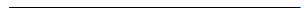Search:

# A dismutation reaction

## Hydrogen peroxide $H_2O_2$ in the presence of a catalyst

### Reaction of hydrogen peroxide and iron(III) chloride:These images are borrowed from the video → Gely

There exist two half-reactions of hydrogen peroxide: (1) $H_2O_2$ $+$ $2e^-$ $+$ $2H^+$ $\longrightarrow$ $2H_2O$ (Product: Water) The oxidation numbers of $O$ vary from $2\cdot(-1)$ $=$ $-2$ to $2\cdot(-2)$ $=$ $-4$, so we have a reduction with gain of $2e^-$: (2) $H_2O_2$ $-$ $2e^-$ $\longrightarrow$ $O_2$ $+$ $2H^+$ (Product: The observed oxygen!) The oxidation numbers of $O$ vary from $2\cdot(-1)$ $=$ $-2$ to $2\cdot0$ $=$ $0$, so we have an oxidation with loss of $2e^-$: The $Fe^{3+}$ ion liberated by iron(III) chloride in water serves as catalyst, i.e. it accelerates the spontaneous reaction! The complete equation: $H_2O_2$ $+$ $2e^-$ $+$ $2H^+$ $\longrightarrow$ $2H_2O$ $H_2O_2$ $-$ $2e^-$ $\longrightarrow$ $O_2$ $+$ $2H^+$$2H_2O_2$ $\longrightarrow$ $2H_2O$ $+$ $O_2$

As hydrogen peroxide is simultaneously reduced and oxidized, it is said to dismutate .